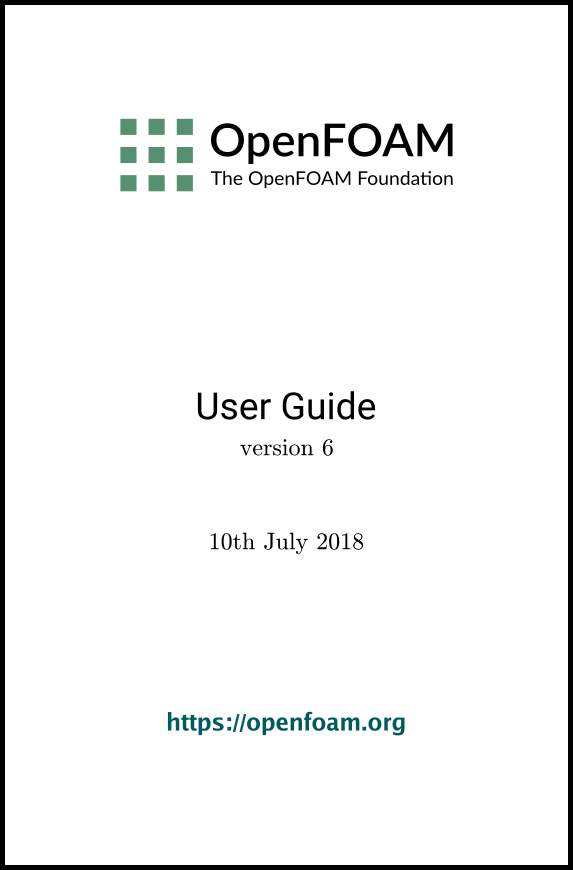## 5.3 Mesh generation with the blockMesh utility

This section describes the mesh generation utility, blockMesh, supplied with OpenFOAM. The blockMesh utility creates parametric meshes with grading and curved edges.

The mesh is generated from a dictionary file named blockMeshDict located in the system (or constant/polyMesh) directory of a case. blockMesh reads this dictionary, generates the mesh and writes out the mesh data to points and faces, cells and boundary files in the same directory.

The principle behind blockMesh is to decompose the domain geometry into a set of 1 or more three dimensional, hexahedral blocks. Edges of the blocks can be straight lines, arcs or splines. The mesh is ostensibly specified as a number of cells in each direction of the block, sufficient information for blockMesh to generate the mesh data.

Each block of the geometry is defined by 8 vertices, one at each corner of a hexahedron. The vertices are written in a list so that each vertex can be accessed using its label, remembering that OpenFOAM always uses the C++ convention that the first element of the list has label ‘0’. An example block is shown in Figure 5.4 with each vertex numbered according to the list. The edge connecting vertices 1 and 5 is curved to remind the reader that curved edges can be specified in blockMesh.

It is possible to generate blocks with less than 8 vertices by collapsing one or more pairs of vertices on top of each other, as described in section 5.3.5.

Each block has a local coordinate system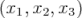that must be right-handed. A right-handed set of axes is defined such that to an observer looking down the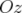axis, with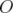nearest them, the arc from a point on the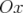axis to a point on the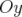axis is in a clockwise sense.

The local coordinate system is defined by the order in which the vertices are presented in the block definition according to:

• the axis origin is the first entry in the block definition, vertex 0 in our example;
• the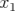direction is described by moving from vertex 0 to vertex 1;
• the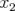direction is described by moving from vertex 1 to vertex 2;
• vertices 0, 1, 2, 3 define the plane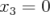;
• vertex 4 is found by moving from vertex 0 in the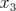direction;
• vertices 5,6 and 7 are similarly found by moving in the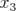direction from vertices 1,2 and 3 respectively.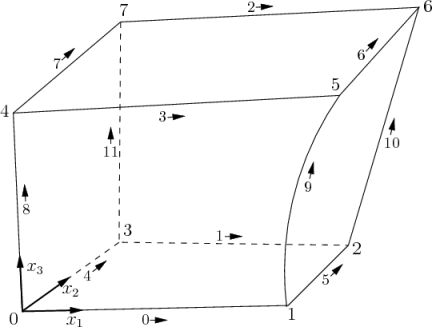Figure 5.4: A single block

Keyword

Description

Example/selection

convertToMeters

Scaling factor for the vertex coordinates

0.001 scales to mm

vertices

List of vertex coordinates

(0 0 0)

edges

Used to describe arc or spline edges

arc 1 4 (0.939 0.342 -0.5)

block

Ordered list of vertex labels and mesh size

 hex (0 1 2 3 4 5 6 7) (10 10 1) simpleGrading (1.0 1.0 1.0)
patches

List of patches

 symmetryPlane base ( (0 1 2 3) )
mergePatchPairs

List of patches to be merged

see section 5.3.2

Table 5.1: Keywords used in blockMeshDict.

### 5.3.1 Writing a blockMeshDict file

The blockMeshDict file is a dictionary using keywords described below.

• convertToMeters: scaling factor for the vertex coordinates, e.g. 0.001 scales to mm.
• vertices: list of vertex coordinates, see section 5.3.1.1.
• edges: used to describe curved geometry, see section 5.3.1.2.
• block: ordered list of vertex labels and mesh size, see section 5.3.1.3.
• boundary: sub-dictionary of boundary patches, see section 5.3.1.5.
• mergePatchPairs: list of patches to be merged, see section 5.3.2.

The convertToMeters keyword specifies a scaling factor by which all vertex coordinates in the mesh description are multiplied. For example,

convertToMeters    0.001;
means that all coordinates are multiplied by 0.001, i.e. the values quoted in the blockMeshDict file are in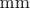.

#### 5.3.1.1 The vertices

The vertices of the blocks of the mesh are given next as a standard list named vertices, e.g. for our example block in Figure 5.4, the vertices are:

vertices
(
( 0    0    0  )    // vertex number 0
( 1    0    0.1)    // vertex number 1
( 1.1  1    0.1)    // vertex number 2
( 0    1    0.1)    // vertex number 3
(-0.1 -0.1  1  )    // vertex number 4
( 1.3  0    1.2)    // vertex number 5
( 1.4  1.1  1.3)    // vertex number 6
( 0    1    1.1)    // vertex number 7
);

#### 5.3.1.2 The edges

Each edge joining 2 vertex points is assumed to be straight by default. However any edge may be specified to be curved by entries in a list named edges. The list is optional; if the geometry contains no curved edges, it may be omitted.

Each entry for a curved edge begins with a keyword specifying the type of curve from those listed in Table 5.2.

 Keyword selection Description Additional entries arc Circular arc Single interpolation point spline Spline curve List of interpolation points polyLine Set of lines List of interpolation points BSpline B-spline curve List of interpolation points line Straight line —

Table 5.2: Edge types available in the blockMeshDict dictionary.

The keyword is then followed by the labels of the 2 vertices that the edge connects. Following that, interpolation points must be specified through which the edge passes. For a arc, a single interpolation point is required, which the circular arc will intersect. For spline, polyLine and BSpline, a list of interpolation points is required. The line edge is directly equivalent to the option executed by default, and requires no interpolation points. Note that there is no need to use the line edge but it is included for completeness. For our example block in Figure 5.4 we specify an arc edge connecting vertices 1 and 5 as follows through the interpolation point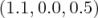:

edges
(
arc 1 5 (1.1 0.0 0.5)
);

#### 5.3.1.3 The blocks

The block definitions are contained in a list named blocks. Each block definition is a compound entry consisting of a list of vertex labels whose order is described in section 5.3, a vector giving the number of cells required in each direction, the type and list of cell expansion ratio in each direction.

Then the blocks are defined as follows:

blocks
(
hex (0 1 2 3 4 5 6 7)    // vertex numbers
(10 10 10)               // numbers of cells in each direction
simpleGrading (1 2 3)    // cell expansion ratios
);
The definition of each block is as follows:
Vertex numbering
The first entry is the shape identifier of the block, as defined in the \$FOAM_ETC-6/cellModels file. The shape is always hex since the blocks are always hexahedra. There follows a list of vertex numbers, ordered in the manner described on page 336.
Number of cells
The second entry gives the number of cells in each of the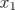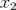and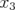directions for that block.
Cell expansion ratios
The third entry gives the cell expansion ratios for each direction in the block. The expansion ratio enables the mesh to be graded, or refined, in specified directions. The ratio is that of the width of the end cell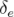along one edge of a block to the width of the start cell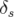along that edge, as shown in Figure 5.5. Each of the following keywords specify one of two types of grading specification available in blockMesh.
The simple description specifies uniform expansions in the local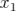,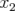and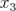directions respectively with only 3 expansion ratios, e.g.
The full cell expansion description gives a ratio for each edge of the block, numbered according to the scheme shown in Figure 5.4 with the arrows representing the direction from first cell…to last cell e.g.
edgeGrading (1 1 1 1 2 2 2 2 3 3 3 3)

This means the ratio of cell widths along edges 0-3 is 1, along edges 4-7 is 2 and along 8-11 is 3 and is directly equivalent to the simpleGrading example given above.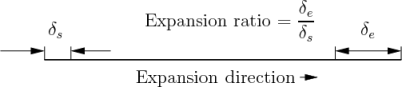Figure 5.5: Mesh grading along a block edge

#### 5.3.1.4 Multi-grading of a block

Using a single expansion ratio to describe mesh grading permits only “one-way” grading within a mesh block. In some cases, it reduces complexity and effort to be able to control grading within separate divisions of a single block, rather than have to define several blocks with one grading per block. For example, to mesh a channel with two opposing walls and grade the mesh towards the walls requires three regions: two with grading to the wall with one in the middle without grading.

OpenFOAM v2.4+ includes multi-grading functionality that can divide a block in an given direction and apply different grading within each division. This multi-grading is specified by replacing any single value expansion ratio in the grading specification of the block, e.g. “1”, “2”, “3” in

blocks
(
hex (0 1 2 3 4 5 6 7) (100 300 100)
);

We will present multi-grading for the following example:

• split the block into 3 divisions in the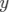-direction, representing 20%, 60% and 20% of the block length;
• include 30% of the total cells in the y-direction (300) in each divisions 1 and 3 and the remaining 40% in division 2;
• apply 1:4 expansion in divisions 1 and 3, and zero expansion in division 2.

We can specify this by replacing the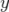-direction expansion ratio “2” in the example above with the following:

blocks
(
hex (0 1 2 3 4 5 6 7) (100 300 100)
(
1                  // x-direction expansion ratio
(
(0.2 0.3 4)    // 20% y-dir, 30% cells, expansion = 4
(0.6 0.4 1)    // 60% y-dir, 40% cells, expansion = 1
(0.2 0.3 0.25) // 20% y-dir, 30% cells, expansion = 0.25 (1/4)
)
3                  // z-direction expansion ratio
)
);

Both the fraction of the block and the fraction of the cells are normalized automatically. They can be specified as percentages, fractions, absolute lengths, etc. and do not need to sum to 100, 1, etc. The example above can be specified using percentages, e.g.

blocks
(
hex (0 1 2 3 4 5 6 7) (100 300 100)
(
1
(
(20 30 4)   // 20%, 30%...
(60 40 1)
(20 30 0.25)
)
3
)
);

#### 5.3.1.5 The boundary

The boundary of the mesh is given in a list named boundary. The boundary is broken into patches (regions), where each patch in the list has its name as the keyword, which is the choice of the user, although we recommend something that conveniently identifies the patch, e.g.inlet; the name is used as an identifier for setting boundary conditions in the field data files. The patch information is then contained in sub-dictionary with:

• type: the patch type, either a generic patch on which some boundary conditions are applied or a particular geometric condition, as listed in section 5.2.1;
• faces: a list of block faces that make up the patch and whose name is the choice of the user, although we recommend something that conveniently identifies the patch, e.g.inlet; the name is used as an identifier for setting boundary conditions in the field data files.

blockMesh collects faces from any boundary patch that is omitted from the boundary list and assigns them to a default patch named defaultFaces of type empty. This means that for a 2 dimensional geometry, the user has the option to omit block faces lying in the 2D plane, knowing that they will be collected into an empty patch as required.

Returning to the example block in Figure 5.4, if it has an inlet on the left face, an output on the right face and the four other faces are walls then the patches could be defined as follows:

boundary               // keyword
(
inlet              // patch name
{
type patch;    // patch type for patch 0
faces
(
(0 4 7 3)  // block face in this patch
);
}                  // end of 0th patch definition

outlet             // patch name
{
type patch;    // patch type for patch 1
faces
(
(1 2 6 5)
);
}

walls
{
type wall;
faces
(
(0 1 5 4)
(0 3 2 1)
(3 7 6 2)
(4 5 6 7)
);
}
);
Each block face is defined by a list of 4 vertex numbers. The order in which the vertices are given must be such that, looking from inside the block and starting with any vertex, the face must be traversed in a clockwise direction to define the other vertices.

When specifying a cyclic patch in blockMesh, the user must specify the name of the related cyclic patch through the neighbourPatch keyword. For example, a pair of cyclic patches might be specified as follows:

left
{
type            cyclic;
neighbourPatch  right;
faces           ((0 4 7 3));
}
right
{
type            cyclic;
neighbourPatch  left;
faces           ((1 5 6 2));
}

### 5.3.2 Multiple blocks

A mesh can be created using more than 1 block. In such circumstances, the mesh is created as has been described in the preceeding text; the only additional issue is the connection between blocks, in which there are two distinct possibilities:

face matching
the set of faces that comprise a patch from one block are formed from the same set of vertices as a set of faces patch that comprise a patch from another block;
face merging
a group of faces from a patch from one block are connected to another group of faces from a patch from another block, to create a new set of internal faces connecting the two blocks.

To connect two blocks with face matching, the two patches that form the connection should simply be ignored from the patches list. blockMesh then identifies that the faces do not form an external boundary and combines each collocated pair into a single internal faces that connects cells from the two blocks.

The alternative, face merging, requires that the block patches to be merged are first defined in the patches list. Each pair of patches whose faces are to be merged must then be included in an optional list named mergePatchPairs. The format of mergePatchPairs is:

mergePatchPairs
(
( <masterPatch> <slavePatch> ) // merge patch pair 0
( <masterPatch> <slavePatch> ) // merge patch pair 1

)
The pairs of patches are interpreted such that the first patch becomes the master and the second becomes the slave. The rules for merging are as follows:
• the faces of the master patch remain as originally defined, with all vertices in their original location;
• the faces of the slave patch are projected onto the master patch where there is some separation between slave and master patch;
• the location of any vertex of a slave face might be adjusted by blockMesh to eliminate any face edge that is shorter than a minimum tolerance;
• if patches overlap as shown in Figure 5.6, each face that does not merge remains as an external face of the original patch, on which boundary conditions must then be applied;
• if all the faces of a patch are merged, then the patch itself will contain no faces and is removed.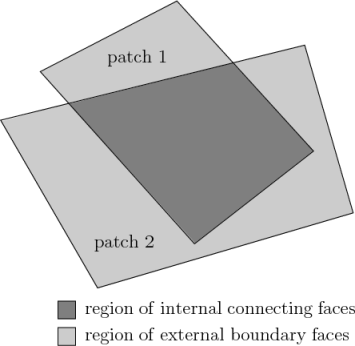Figure 5.6: Merging overlapping patches

The consequence is that the original geometry of the slave patch will not necessarily be completely preserved during merging. Therefore in a case, say, where a cylindrical block is being connected to a larger block, it would be wise to the assign the master patch to the cylinder, so that its cylindrical shape is correctly preserved. There are some additional recommendations to ensure successful merge procedures:

• in 2 dimensional geometries, the size of the cells in the third dimension, i.e. out of the 2D plane, should be similar to the width/height of cells in the 2D plane;
• it is inadvisable to merge a patch twice, i.e. include it twice in mergePatchPairs;
• where a patch to be merged shares a common edge with another patch to be merged, both should be declared as a master patch.

### 5.3.3 Projection of vertices, edges and faces

blockMesh can be configured to create body fitted meshes using projection of vertices, edges and/or faces onto specified geometry. The functionality can be used to mesh spherical and cylindrical geometries such as pipes and vessels conveniently. The user can specify within the blockMeshDict file within an optional geometry dictionary with the same format as used in the snappyHexMeshDict file. For example to specify a cylinder using the built in geometric type the user could configure with the following:

geometry
{
cylinder
{
type searchableCylinder;
point1 (0 -4 0);
point2 (0 4 0);
}
};

The user can then project vertices, edges and/or faces onto the cylinder surface with the project keyword using example syntax shown below:

vertices
(
project (-1 -0.1 -1) (cylinder)
project ( 1 -0.1 -1) (cylinder)
...
);

edges
(
project 0 1 (cylinder)
...
);

faces
(
project (0 4 7 3) cylinder
...
);

The use of this functionality is demonstrated in tutorials which can be located by searching for the project keyword in all the blockMeshDict files by:

find \$FOAM_TUTORIALS -name blockMeshDict | xargs grep -l project

### 5.3.4 Naming vertices, edges, faces and blocks

Vertices, edges, faces and blocks can be named in the configuration of a blockMeshDict file, which can make it easier to manage more complex examples. It is done simply using the name keyword. The following syntax shows naming using the example for projection in the previous subsection:

vertices
(
name v0 project (-1 -0.1 -1) (cylinder)
name v1 project ( 1 -0.1 -1) (cylinder)
...
);

edges
(
project v0 v1 (cylinder)
...
);

When a name is provided for a given entity, it can be used to replace the index. In the example about, rather than specify the edge using vertex indices 0 and 1, the names v0 and v1 are used.

### 5.3.5 Creating blocks with fewer than 8 vertices

It is possible to collapse one or more pair(s) of vertices onto each other in order to create a block with fewer than 8 vertices. The most common example of collapsing vertices is when creating a 6-sided wedge shaped block for 2-dimensional axi-symmetric cases that use the wedge patch type described in section 5.2.2. The process is best illustrated by using a simplified version of our example block shown in Figure 5.7. Let us say we wished to create a wedge shaped block by collapsing vertex 7 onto 4 and 6 onto 5. This is simply done by exchanging the vertex number 7 by 4 and 6 by 5 respectively so that the block numbering would become:

hex (0 1 2 3 4 5 5 4)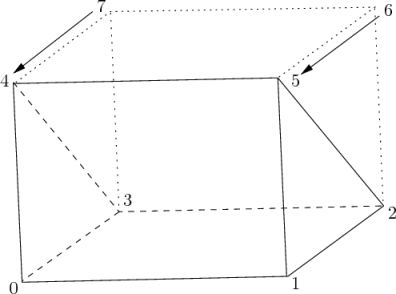Figure 5.7: Creating a wedge shaped block with 6 vertices

The same applies to the patches with the main consideration that the block face containing the collapsed vertices, previously (4 5 6 7) now becomes (4 5 5 4). This is a block face of zero area which creates a patch with no faces in the polyMesh, as the user can see in a boundary file for such a case. The patch should be specified as empty in the blockMeshDict and the boundary condition for any fields should consequently be empty also.

### 5.3.6 Running blockMesh

As described in section 3.3, the following can be executed at the command line to run blockMesh for a case in the <case> directory:

blockMesh -case <case>
The blockMeshDict file must exist in the system (or constant/polyMesh) directory.
OpenFOAM v6 User Guide - 5.3 Mesh generation with the blockMesh utility